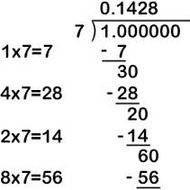# Decimal computation by hand

• B
• Mr Davis 97

#### Mr Davis 97

I have to perform the following computation by hand to get a number within two decimal places:
##\displaystyle (120)(\frac{54.30}{194.79})##. The answer is 33.45, but how can I do this by hand?

I have to perform the following computation by hand to get a number within two decimal places:
##\displaystyle (120)(\frac{54.30}{194.79})##. The answer is 33.45, but how can I do this by hand?
Well, the multiplication part should be easy enough. It's the long division which takes a bit more effort.

Remember, $$a ⋅ \frac{b}{c} = \frac{a ⋅ b}{c}$$

Don't tell me you've never done any arithmetic by hand.

multiply top and bottom by 1 ie 100/100 to get 120 * 5430/19479

next use long division

You could first multiply the numerator by 120 before you divide

Okay, so doing the long division ##\frac{651600}{19479}## I can get to 33, but then I have the remainder ##\frac{8793}{19479}##. How can I figure out that this remainder is approximately 0.45?

Have you forgotten how to do long division?

Here's an example image: